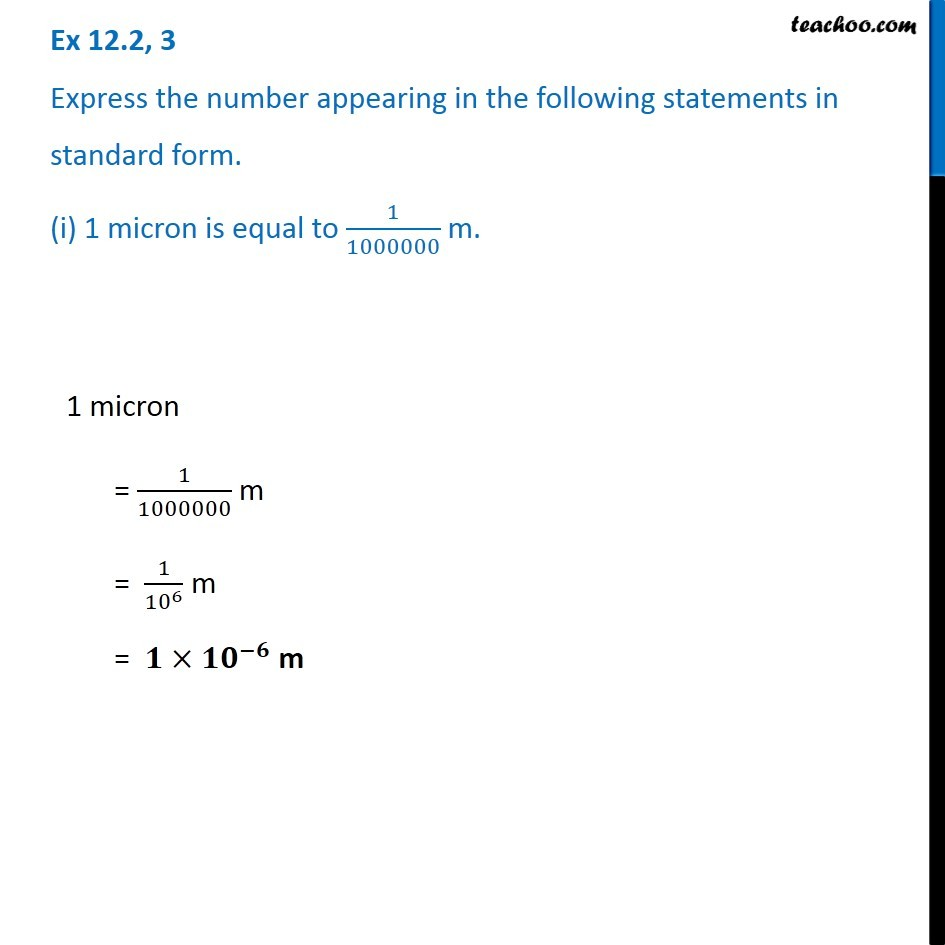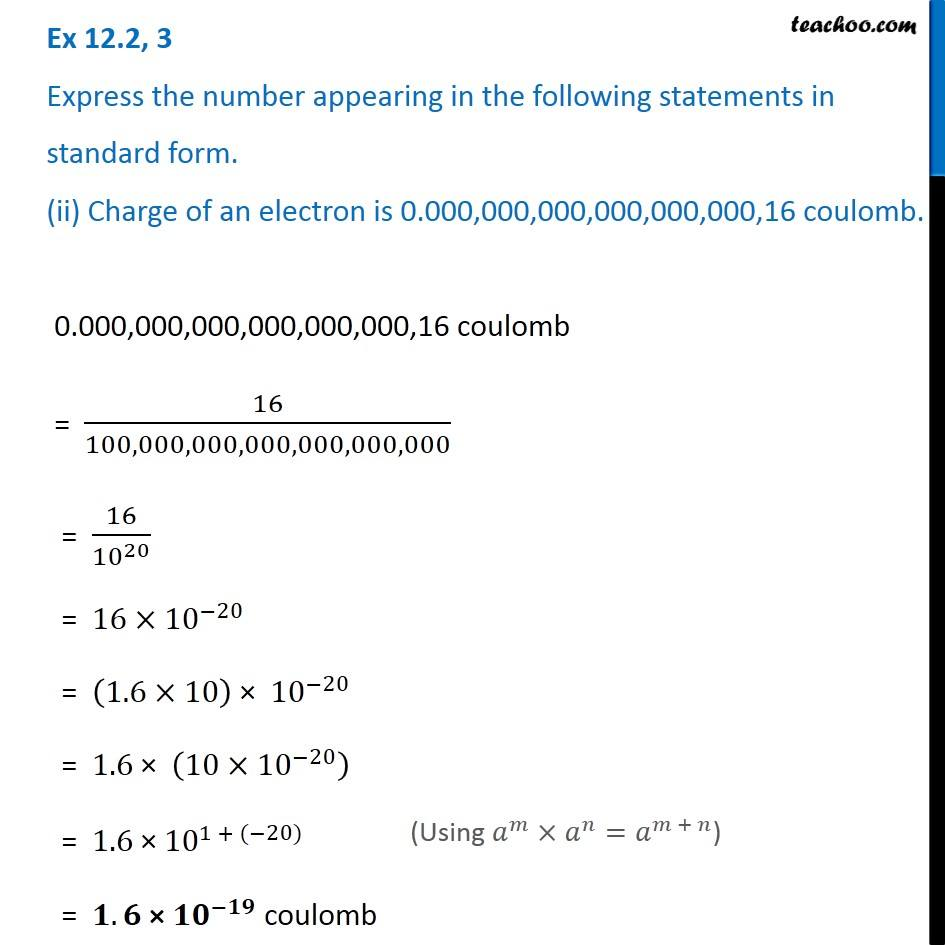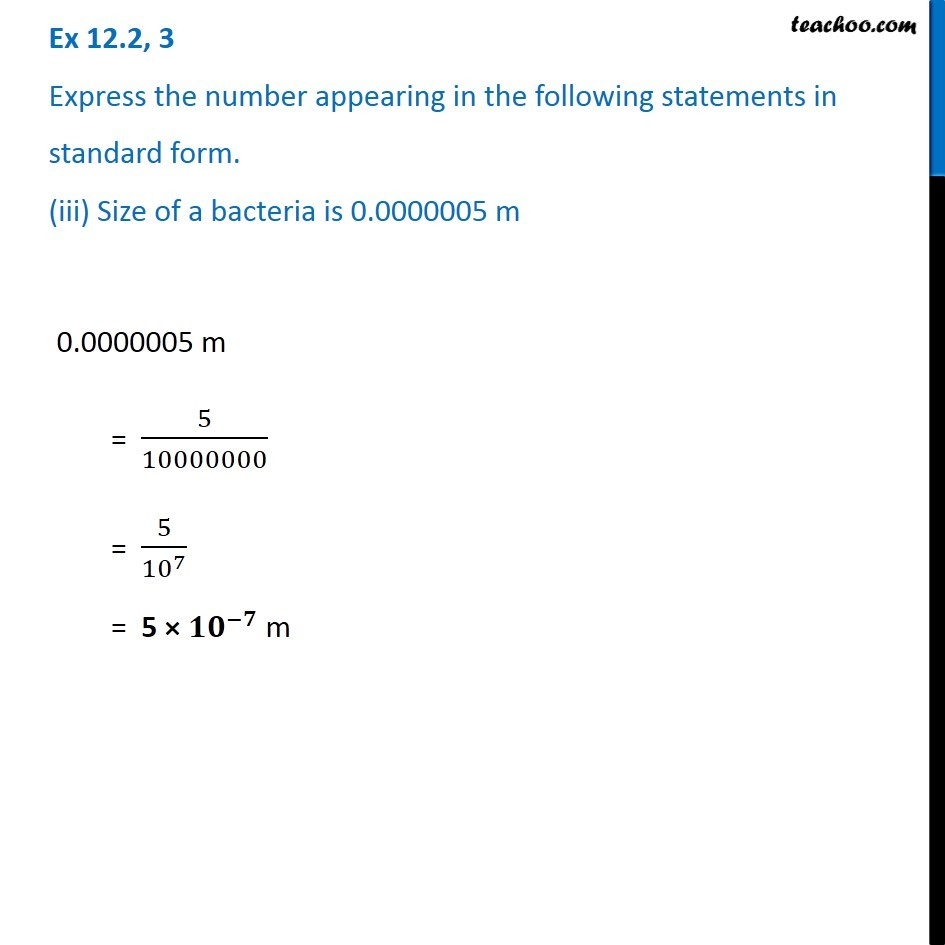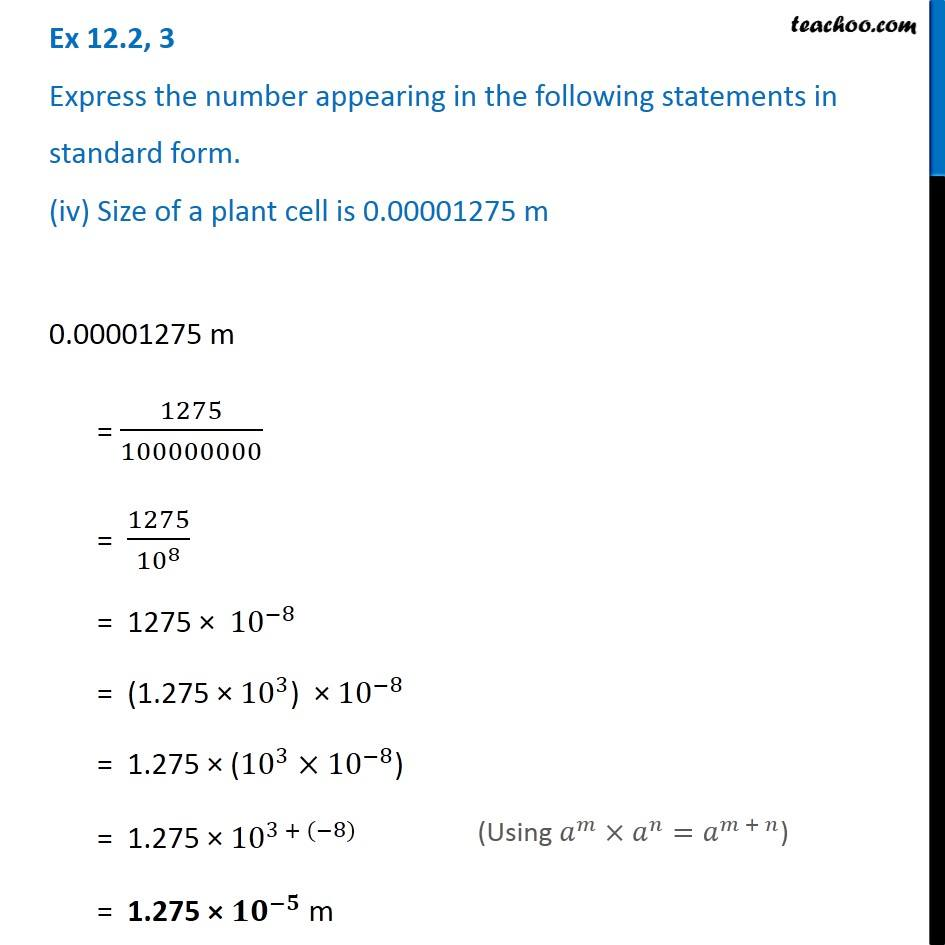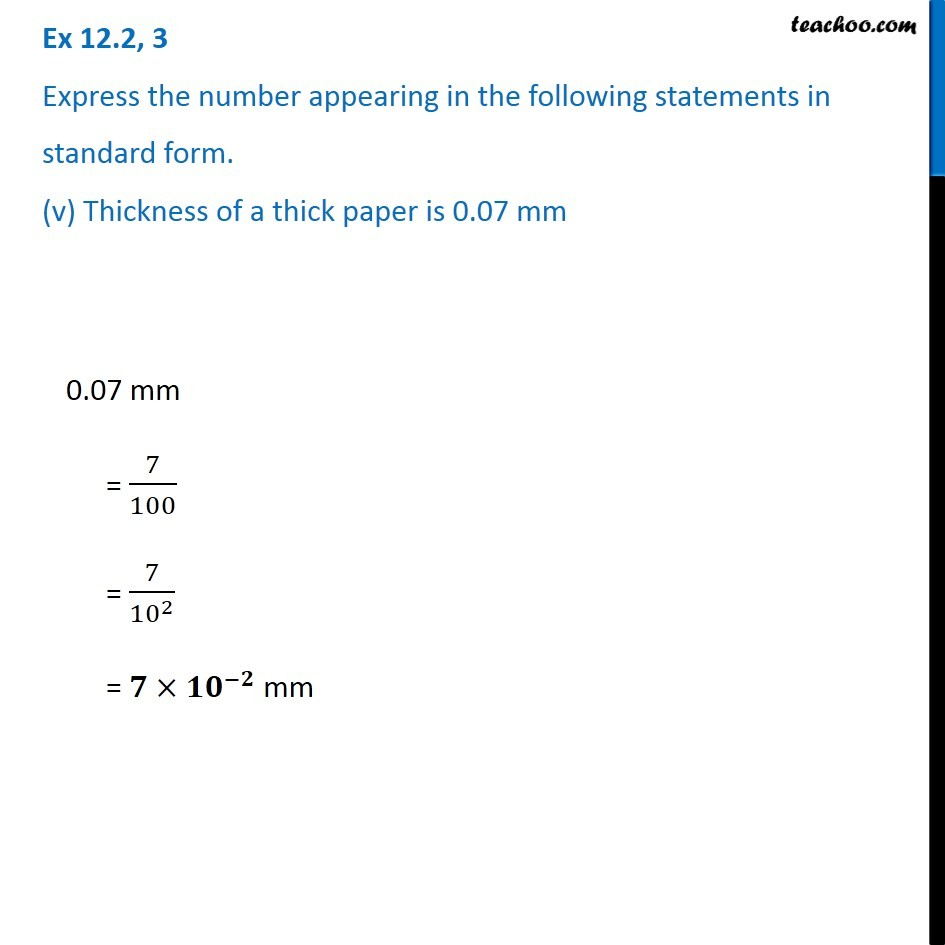Subscribe to our Youtube Channel - https://you.tube/teachoo

1. Chapter 12 Class 8 Exponents and Powers
2. Serial order wise
3. Ex 12.2

Transcript

Ex 12.2, 3 Express the number appearing in the following statements in standard form. (i) 1 micron is equal to 1/1000000 m. 1 micron = 1/1000000 m = 1/〖10〗^6 m = 𝟏×〖𝟏𝟎〗^(−𝟔) m Ex 12.2, 3 Express the number appearing in the following statements in standard form. (ii) Charge of an electron is 0.000,000,000,000,000,000,16 coulomb. 0.000,000,000,000,000,000,16 coulomb = 16/100,000,000,000,000,000,000 = 16/〖10〗^20 = 16×〖10〗^(−20) = (1.6×10) × 〖10〗^(−20) = 1.6 × (10×〖10〗^(−20) ) = 1.6 × 〖10〗^(1 + (−20)) = 𝟏.𝟔 × 〖𝟏𝟎〗^(−𝟏𝟗) coulomb (Using 𝑎^𝑚×𝑎^𝑛=𝑎^(𝑚 + 𝑛)) Ex 12.2, 3 Express the number appearing in the following statements in standard form. (iii) Size of a bacteria is 0.0000005 m 0.0000005 m = 5/10000000 = 5/〖10〗^7 = 5 × 〖𝟏𝟎〗^(−𝟕) m Ex 12.2, 3 Express the number appearing in the following statements in standard form. (iv) Size of a plant cell is 0.00001275 m 0.00001275 m = 1275/100000000 = 1275/〖10〗^8 = 1275 × 〖10〗^(−8) = (1.275 × 〖10〗^3) × 〖10〗^(−8) = 1.275 × (〖10〗^3×〖10〗^(−8)) = 1.275 × 〖10〗^(3 + (−8)) = 1.275 × 〖𝟏𝟎〗^(−𝟓) m (Using 𝑎^𝑚×𝑎^𝑛=𝑎^(𝑚 + 𝑛)) Ex 12.2, 3 Express the number appearing in the following statements in standard form. (v) Thickness of a thick paper is 0.07 mm 0.07 mm = 7/100 = 7/〖10〗^2 = 𝟕×〖𝟏𝟎〗^(−𝟐) mm

Ex 12.2